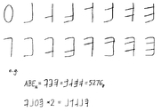xOverview

In mathematics
Mathematics
Mathematics is the study of quantity, space, structure, and change. Mathematicians seek out patterns and formulate new conjectures. Mathematicians resolve the truth or falsity of conjectures by mathematical proofs, which are arguments sufficient to convince other mathematicians of their validity...

and computer science
Computer science
Computer science or computing science is the study of the theoretical foundations of information and computation and of practical techniques for their implementation and application in computer systems...

In mathematical numeral systems, the base or radix for the simplest case is the number of unique digits, including zero, that a positional numeral system uses to represent numbers. For example, for the decimal system the radix is ten, because it uses the ten digits from 0 through 9.In any numeral...

, or hex) is a positional
Positional notation
Positional notation or place-value notation is a method of representing or encoding numbers. Positional notation is distinguished from other notations for its use of the same symbol for the different orders of magnitude...

numeral system
Numeral system
A numeral system is a writing system for expressing numbers, that is a mathematical notation for representing numbers of a given set, using graphemes or symbols in a consistent manner....

In mathematical numeral systems, the base or radix for the simplest case is the number of unique digits, including zero, that a positional numeral system uses to represent numbers. For example, for the decimal system the radix is ten, because it uses the ten digits from 0 through 9.In any numeral...

, or base, of 16. It uses sixteen distinct symbols, most often the symbols 09 to represent values zero to nine, and A, B, C, D, E, F (or alternatively af) to represent values ten to fifteen. For example, the hexadecimal number 2AF3 is equal, in decimal, to (2 × 163) + (10 × 162) + (15 × 161) + (3 × 160), or 10,995.

Each hexadecimal digit represents four binary digits (bit
Bit
A bit is the basic unit of information in computing and telecommunications; it is the amount of information stored by a digital device or other physical system that exists in one of two possible distinct states...

s) (also called a "nibble
Nibble
In computing, a nibble is a four-bit aggregation, or half an octet...

"), and the primary use of hexadecimal notation is as a human-friendly representation of binary-coded
Binary code
A binary code is a way of representing text or computer processor instructions by the use of the binary number system's two-binary digits 0 and 1. This is accomplished by assigning a bit string to each particular symbol or instruction...

values in computing and digital electronics.